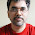## Thursday, March 20, 2014

### Background

In the forth coming week, I will be giving a presentation on the fundamentals of imputation to my colleagues. One of the most important idea I would like to present is multiple imputation.

In my last post, I have given a small example of multiple imputation, but it does not provide the evidence why we should use it. This is the aim of this post in which I have taken a small example to illustrate how inference may change under single and multiple imputation.

Like most international organization and statistical institutes, we use the least-squares growth rate whenever possible to estimate the growth of a certain indicator. In brevity, a linear regression on the logged variable with respect to time is fitted, then the exponential of the time coefficient minus one will be the growth rate. $LSGR = (e^{\hat{\beta}} - 1) \times 100$

Since a linear regression is fitted, we can conveniently test whether a growth exist. In this example, I have chosen the production of wheat of Romania to illustrate my point of why multiple imputation should be used for drawing inference and to avoid being over confident.

### Method

Lets load the data and simulate some missing value.



## Load the data, the production domain of Romania
romaniaMaize.df = structure(list(
year = 1961:2011,
production = c(5739600, 4932400, 6022700, 6691700,
5877000, 8021600, 6857879, 7105287, 7675808, 6535512,
7850300, 9816700, 7397200, 7439600, 9240661,
11583200, 10113559, 9671200, 11768100, 10563300,
8321500, 10550000, 10296000, 9891000, 11903200,
10900900, 7526900, 7182200, 6761800, 6809604,
10497338, 6828270, 7987450, 9343000, 9923132,
9607944, 12686700, 8623370, 10934800, 4897600,
9119200, 8399800, 9576985, 14541564, 10388499,
8984729, 3853920, 7849080, 7973258, 9042032,
11717591),
areaHarvested = c(3428400, 3106766, 3379300, 3319100,
3305800, 3287700, 3221075, 3344013, 3293068,
3084036, 3131400, 3196500, 2956800, 2963000,
3304691, 3377600, 3317671, 3178798, 3311291,
3287560, 3077900, 2764387, 2935120, 3090530,
3090100, 2858100, 2787100, 2579400, 2733400, 2466735,
2574999, 3335920, 3065682, 2983400, 3109236, 3277041,
3037700, 3128900, 3013400, 3049400, 2974000, 2761223,
3119104, 3196130, 2609110, 2512944, 2263080, 2432210,
2333501, 2094249, 2587102),
seed = c(124000, 135000, 133000, 132000, 139000, 137000,
140000, 140000, 135000, 135000, 138000, 135000,
133000, 140000, 120000, 107000, 134000, 139000,
133000, 135000, 124000, 144000, 148000, 145000,
135000, 130000, 122000, 130000, 139000, 145000,
180000, 180000, 142500, 125600, 130900, 90587,
124753, 77000, 76000, 69907, 70692, 72785, 79065,
67000, 64600, 63829, 65123, 62851, 59985, 53880,
64744)),
.Names = c("year", "production", "areaHarvested", "seed"),
row.names = 6110:6160, class = "data.frame")

## Simulate missing value in production
n = NROW(romaniaMaize.df)
pctMiss = 0.5
myseed = 587
set.seed(myseed)
missIndex = sample(n, n * pctMiss)
romaniaMaize.df$productionSim = romaniaMaize.df$production
romaniaMaize.df[missIndex, "productionSim"] = NA



Then I will impute the data using the mi package assuming that production depends on the seed utilized and area harvested. I have imputed the data 50 times for the plot, but according to Rubin 3 to 10 imputation would be sufficient.



## Create missing information matrix
info = mi.info(romaniaMaize.df)
info = mi.info.update.include(info,
list(production = FALSE, year = TRUE))

## I assume that the production has a trend and is determined by the
## area harvested and seed utilized.
info = mi.info.update.imp.formula(info,
list(productionSim = "productionSim ~ year + areaHarvested + seed"))

## multiple imputation via mi
romaniaMaize.mi = mi(romaniaMaize.df, info = info, n.imp = 50,
n.iter = 100, seed = myseed, run.past.convergence = TRUE)



Then we compute the single imputation by taking the average value of all the multiple imputation.


## Compute the single imputation
romaniaMaize.df$imputed = romaniaMaize.df$productionSim
romaniaMaize.df[is.na(romaniaMaize.df$imputed), "imputed"] = rowMeans(sapply(romaniaMaize.mi@imp, FUN = function(chain) chain$productionSim@random))



The following plot illustrate the difference between multiple and single imputation. For single imputation we impute only once, while in multiple imputation we impute multiple times to reflect the uncertainty, each set of imputation can be interpreted as a potentially observed realization.



## Plot and compare the imputations
with(romaniaMaize.df, plot(year, production, type = "n",
ylim = c(0, max(production, imputed))))
sapply(mi.completed(romaniaMaize.mi), FUN = function(chain)
lines(romaniaMaize.df$year, chain$productionSim,
col = rgb(0.5, 0.5, 0.5, alpha = 0.3)))
with(romaniaMaize.df, lines(year, production,
ylim = c(0, max(production, imputed))))
with(romaniaMaize.df, points(year, production, pch = 19,
ylim = c(0, max(production, imputed)),
col = is.na(productionSim) + 1))
with(romaniaMaize.df, lines(year, imputed, col = "steelblue", lwd = 2))



Compute the growth rate on single and multiple imputation and test using the frequentists approach.


> ## Fit the regression on single/multiple imputed data set
> single.fit = lm(log(romaniaMaize.df$imputed) ~ year, + data = romaniaMaize.df) > multiple.fit = lm.mi(log(productionSim) ~ year, romaniaMaize.mi) > > ## Compare the growth rate > (exp(coef(single.fit)) - 1) * 100 year 0.59327 > ((exp(coef(multiple.fit)) - 1) * 100) year 0.6079975 > ## Compare the P-value > 2 * pt(single.fit$coef/sqrt(diag(vcov(single.fit))),
+        df = single.fit$df.residual, lower.tail = FALSE) year 4.187208e-05 > 2 * pt(multiple.fit@mi.pooled$coef/multiple.fit@mi.pooled$se, + df = single.fit$df.residual, lower.tail = FALSE)
year
0.05538677


From the result we can see that the point estimates are very similar. However, if we perform the hypothesis testing on the singularly imputed dataset, we would have came to the conclusion that the production of Romania has been growing at a rate which is statistical significant over the past half a century. On the other hand, the test on the multiplied dataset has a P-value greater than the typical threshold of 5 percent.

Why are the results different? Lets not get into all the issues of the hypothesis testing under the Frequentist paradigm. The main disparity results from the fact that we take into account of the uncertainty of imputation and correctly estimate the standard error. Rather than providing a single point we provide a distribution of possible points.

1.I am using mi (Version 0.09-18.03, built: 2013-8-22).

This works :
> info = mi.info(romaniaMaize.df)

But after that:
> info = mi.info.update.include(info,
+ list(areaCode = FALSE, unsdSubReg = FALSE, production = FALSE,
+ year = TRUE))
Error in object[[jj]]$imp.formula :$ operator is invalid for atomic vectors

2.I have updated the code, the columne "areaCode", and "unsdSubReg" were in my original analysis but not anymore.